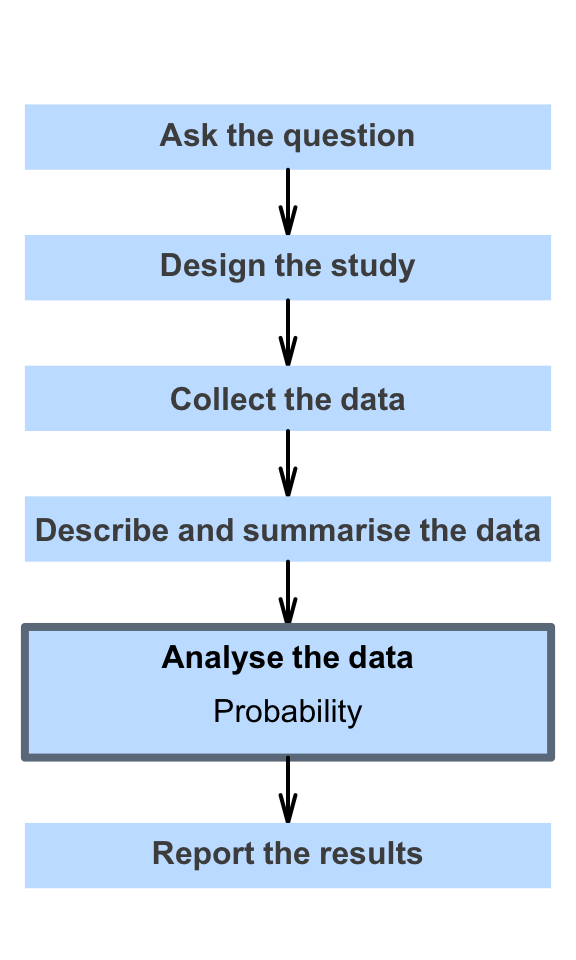# 16 Probability

So far, you have learnt to ask a RQ, identify different ways of obtaining data, design the study, collect the data describe the data, summarise data graphically and numerically, and understand the decision-making process.

In this chapter, you will learn about probability to describe the random nature of sample statistics. You will learn to:

• explain probabilities.
• apply the classical approach to probability in simple situations.
• apply the relative frequency approach to probability in simple situations.
• apply the subjective approach to probability in simple situations.
• identify events that are independent.